Monday 08th August 2022

# AIPMT 2007 Physics Screening

Q. 1. Dimensions of resistance in an electrical circuit, in terms of dimension of mass M, of length L, of time T and of current I, would be

1.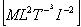2.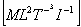3.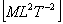4.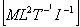Sol.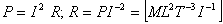Correct choice: (1)

Q. 2. A particle moving along x-axis has acceleration f, at time t, given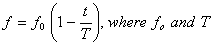are constant. The particle at t = 0 has zero velocity. In the time interval between t = 0 and the instant when f = 0 , the particle’s velocity (vx ) is

1.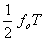2.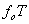3.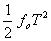4.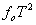Sol.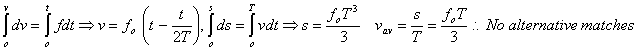If they have asked instantaneous velocity when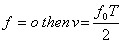Correct choice: (1)

Q. 3. A car moves from X to Y with a uniform speed vu and returns to Y with a uniform speed vd .The average speed for this round trip is

1.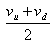2.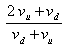3.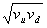4.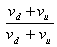Sol. In question it must be car moves from X to Y and returns to X.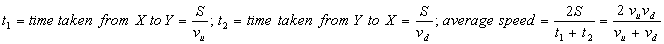Correct choice: (2)

Q. 4. A particle starting from the origin (0, 0) moves in a straight line in the (x, y) plane. Its co-ordinates at a later time are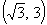. The path of the particle makes with the x-axis an angle of

1.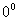2.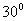3.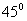4.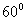Sol.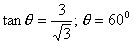Correct choice: (4)

Q. 5. A block B is pushed momentarily along a horizontal surface with an initial velocity V. If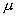is the coefficient of sliding friction between B and the surface, block B will come to rest after a time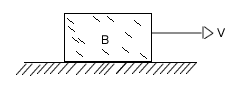1.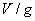2.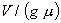3.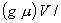4.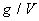Sol.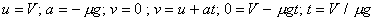Correct choice: (2)

Q. 6. A vertical spring with force constant K is fixed on a table. A ball of mass m at a height h above the free upper end of the spring falls vertically on the spring so that the spring is compressed by a distance d . The net work done in the process is

1.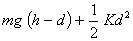2.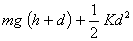3.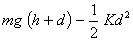4.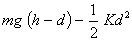Sol.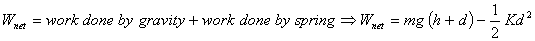Correct choice: (3)

Q. 7. A wheel has angular acceleration of 3.0 rad/sec2 and an initial angular speed of 2.00 rad / sec. In a time of 2 sec it has rotated through an angle (in radian) of

1. 4
2. 6
3. 10
4. 12

Sol.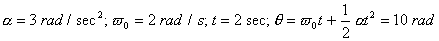Correct choice: (3)

Q. 8.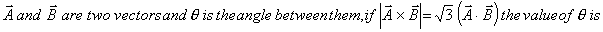1.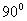2.3.4.Sol.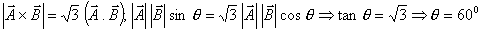Correct choice: (2)

Q. 9. The position x of a particle with respect to time t along x -axis is given by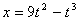where x is in metres and t in second. What will be the position of this particle when it achieves maximum speed along the +x direction?

1. 24 m
2. 32 m
3. 54 m
4. 81 m

Sol.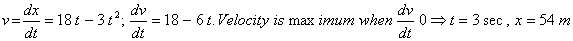Correct choice: (3)

Q. 10. A mass of 2.0 kg is put on a flat pan attached to a vertical spring fixed on the ground as shown in the figure. The mass of the spring and the pan is negligible. When pressed slightly and released the mass executes a simple harmonic motion. The spring constant is 200 N/m. What should be the minimum amplitude of the motion so that the mass gets detached from the pan?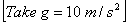1. 4.0 cm
2. 8.0 cm
3. 10.0 cm
4. any value less than 12.0 cm

Sol.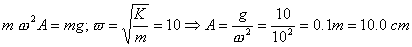Correct choice: (3)

Q. 11. A particle of mass m moves in the XY plane with a velocity V along the straight line AB. If the angular momentum of the particle with respect to origin O is LA when it is at A and LB when it is at B, then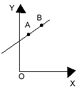1.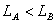2.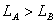3.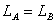4. The relationship between LA and LB depends upon the slope of the line AB

Sol.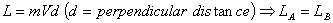Correct choice: (3)

Q. 12. A uniform rod AB of length l and mass m is free to rotate about point A. The rod is released from rest in the horizontal position. Given that the moment of inertia of the rod about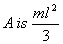, the initial angular acceleration of the rod will be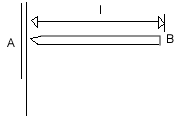1.2.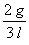3.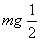4.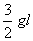Sol.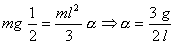Correct choice: (1)

Q. 13. Two satellites of earth,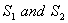are moving in the same orbit. The mass of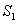is four times the mass of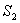. Which one of the following statements is true?

1. The kinetic energies of the two satellites are equal.
2. The time period of isfour times that of.
3. The potential energies of earth and satellite in the two cases are equal.
4.are moving with the same speed.

Sol . As orbital velocity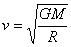it is independent of mass of satellite Correct choice: (4)

Q. 14. Assuming the sun to have a spherical outer surface of radius r , radiating like a black body at temperature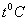, the power received by a unit surface, (normal to the incident rays) at a distance R from the center of the sun is (where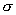is the Stefan’s constant).

1.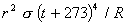2.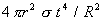3.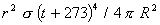4.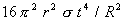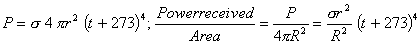Correct choice: (1)

Q. 15. An engine has an efficiency of 1/6. When the temperature of sink is reduced by 620C, its efficiency is doubled. Temperature of the source is

1.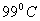2.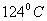3.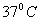4.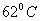Sol.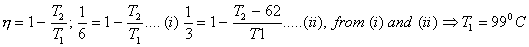Correct choice: (1)

Q. 16. A black body is at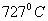. It emits energy at a rate which is proportional to

1.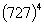2.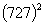3.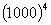4.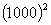Sol.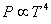Correct choice: (3)

Q. 17. The frequency of a light wave in a material is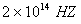and wavelength is 5000Å. The refractive index of material will be

1. 1.33
2. 1.40
3. 1.50
4. 3.00

Sol.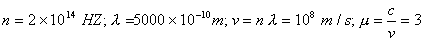Correct choice: (4)

Q. 18. The phase difference between the instantaneous velocity and acceleration of a particle executing simple harmonic motion is

1. (1) zero
2.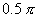3.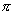4.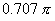Sol.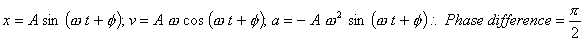Correct choice: (2)

Q. 19. The particle executing simple harmonic motion has a kinetic energy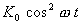. The maximum values of the potential energy and the total energy are respectively

1.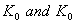2.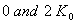3.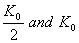4.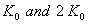Sol.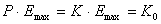Correct choice: (1)

Q. 20. A particle executes simple harmonic oscillation with an amplitude a . The period of oscillation is T. The minimum time taken by the particle to travel half of the amplitude from the equilibrium position is

1. T / 2
2. T / 4
3. T / 8
4. T /12

Sol.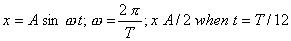Correct choice: (4)